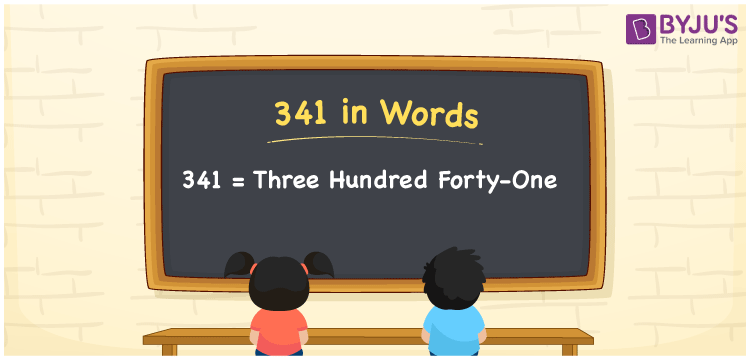# 341 in words

341 in words is written as Three Hundred and Forty One. 341 represents the count or value. The article on Counting Numbers can give you an idea about count or counting. The number 341 is a 3 digit number that is used in expressions related to money, days, distance, length, weight and so on. Let us consider an example for 341. “As a hobby, I have collected Three Hundred and Forty One stamps till date”.

 341 in words Three Hundred and Forty One Three Hundred and Forty One in Numbers 341

## 341 in English Words## How to Write 341 in Words?

We can convert 341 to words using a place value chart. The number 341 has 3 digits, so let’s make a chart that shows the place value up to 3 digits.

 Hundreds Tens Ones 3 4 1

Thus, we can write the expanded form as:

3 × Hundred + 4 × Ten + 1 × One

= 3 × 100 + 4 × 10 + 1 × 1

= 341

= Three Hundred and Forty One.

341 is the natural number that is succeeded by 340 and preceded by 342.

341 in words – Three Hundred and Forty One.

Is 341 an odd number? – Yes.

Is 341 an even number? – No.

Is 341 a perfect square number? – No.

Is 341 a perfect cube number? – No.

Is 341 a prime number? – No.

Is 341 a composite number? – Yes.

## Solved Example

1. Write the number 341 in expanded form

Solution: 3 × 100 + 4 × 10 + 1 × 1

We can write 341 = 300 + 40 + 1

= 3 × 100 + 4 × 10 + 1 × 1.

## Frequently Asked Questions on 341 in words

Q1

### How to write the number 341 in words?

341 in words is written as Three Hundred and Forty One.
Q2

### Is 341 divisible by 3?

No. 341 is not divisible by 3.
Q3

### Is 341 a prime number?

No. 341 is not a prime number.